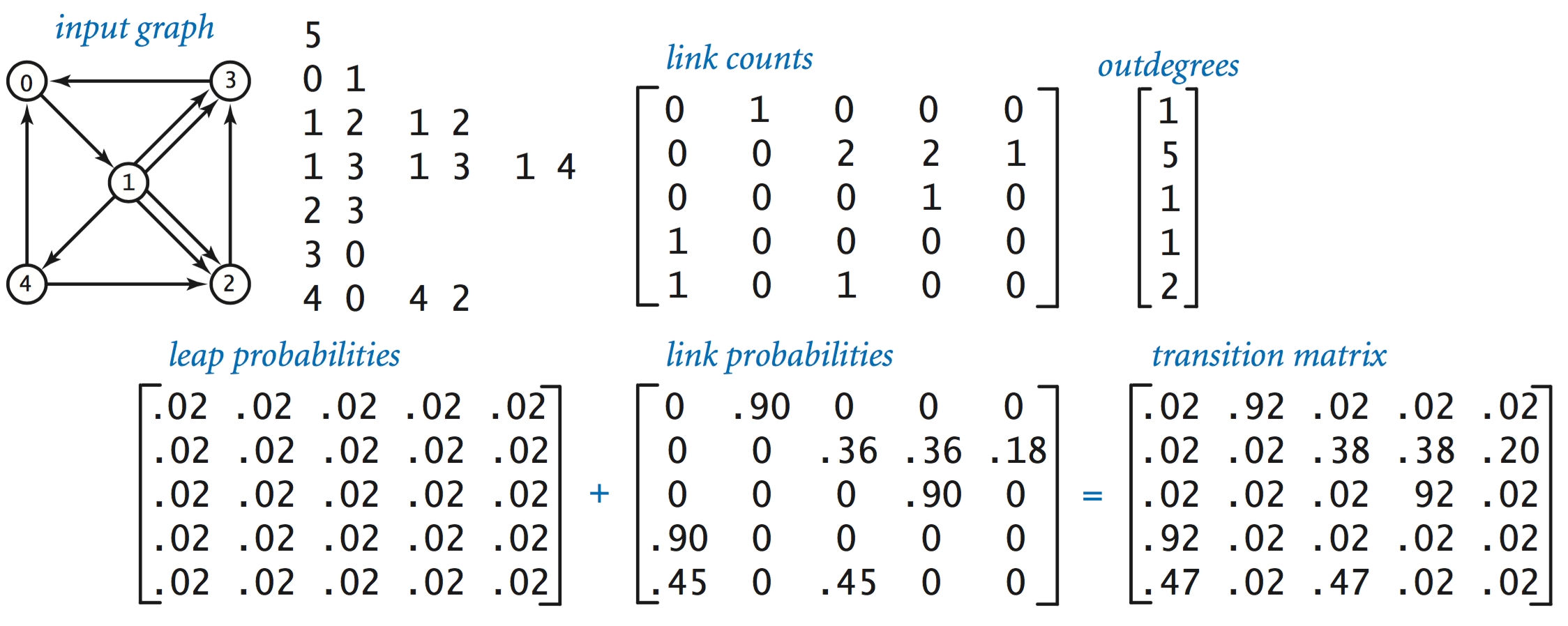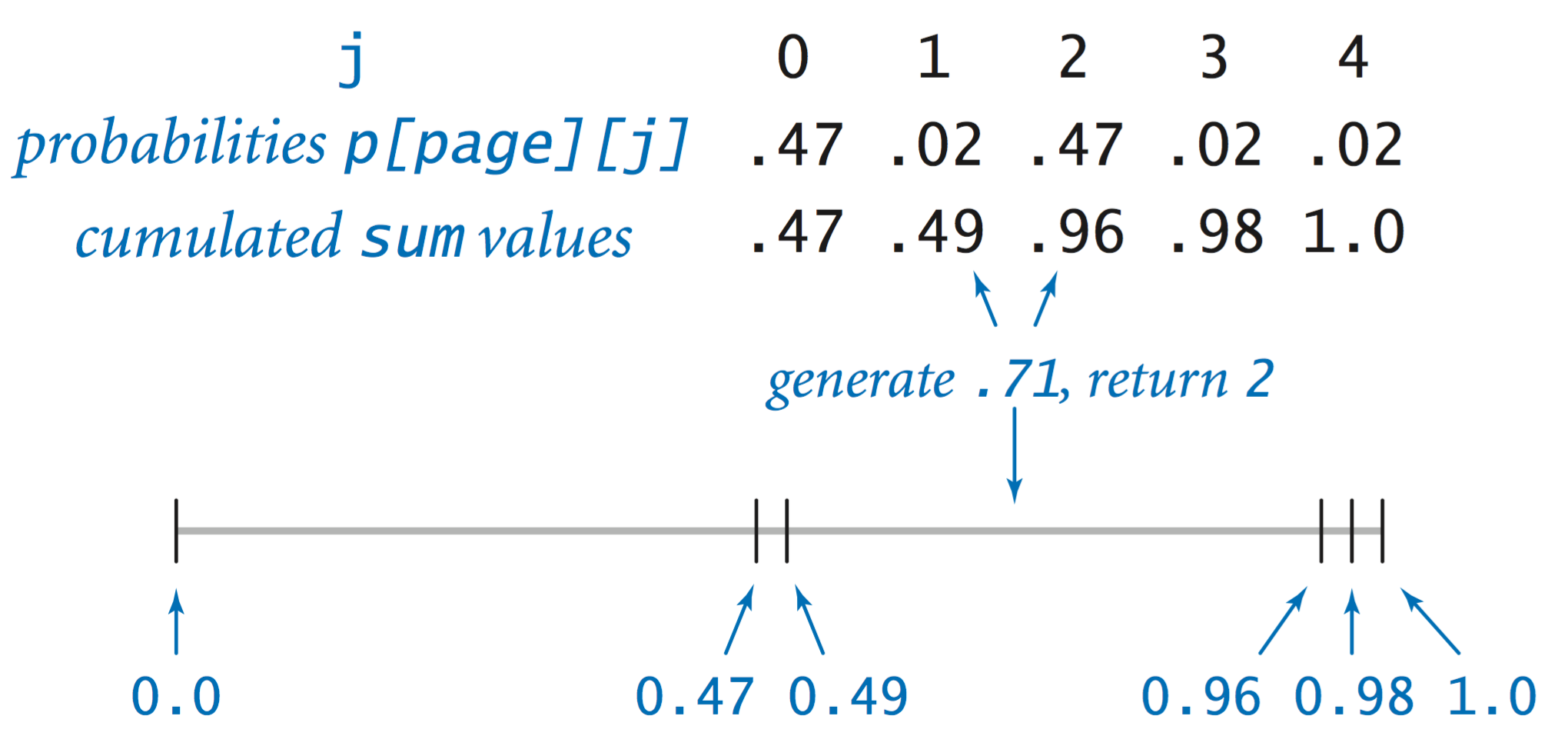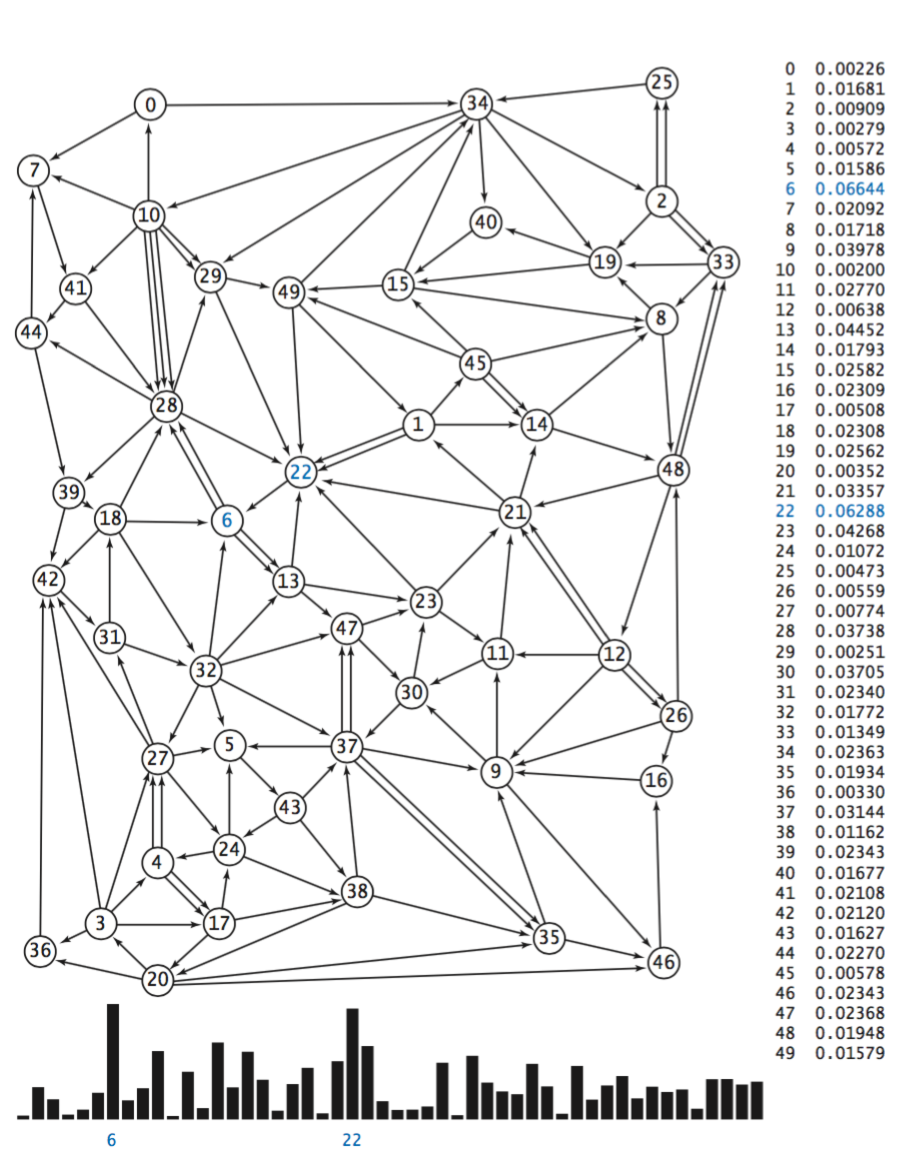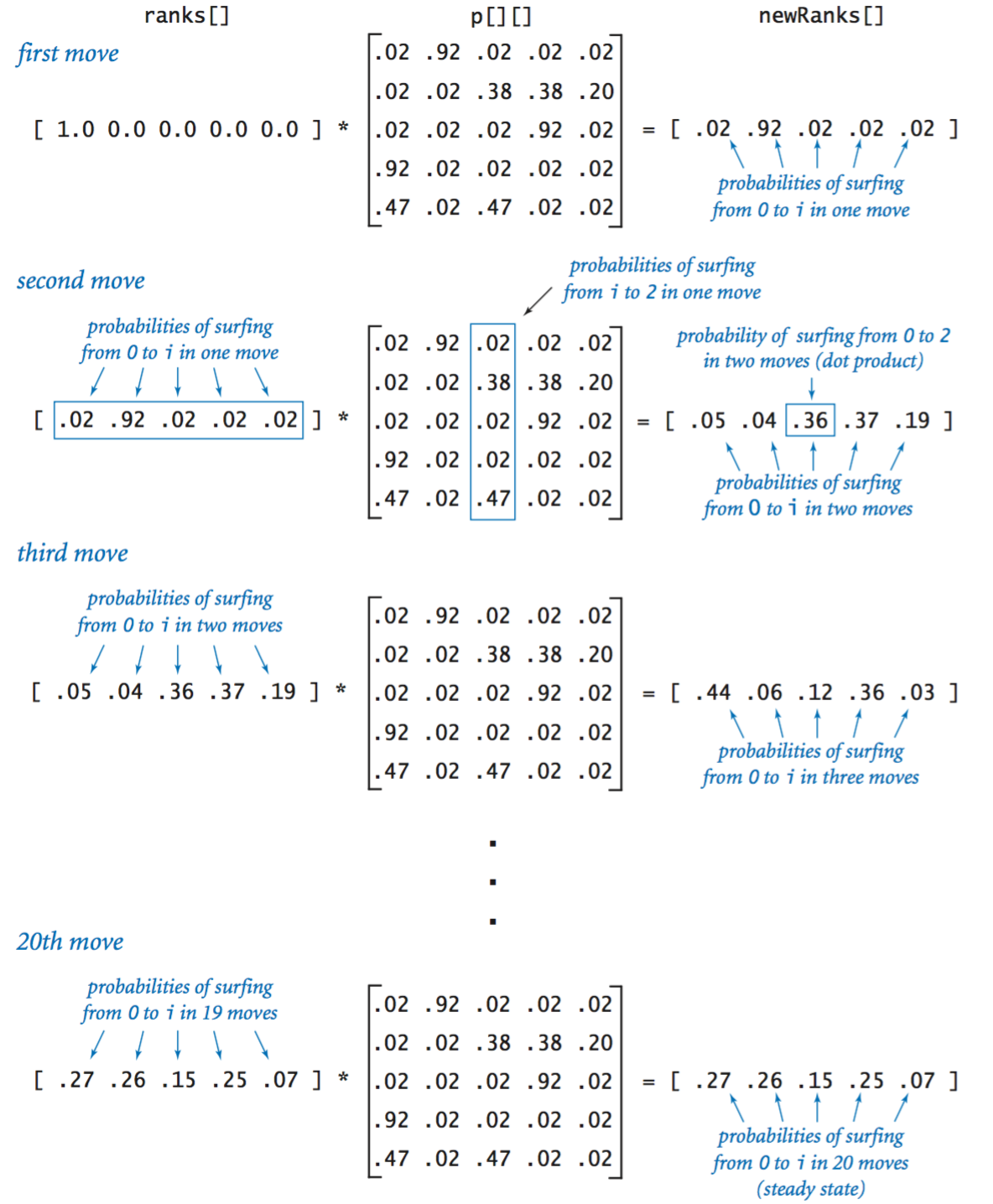# 1.6   Case Study:   Random Web Surfer

Communicating across the web has become an integral part of everyday life. This communication is enabled in part by scientific studies of the structure of the web. We consider a simple model, known as the random-surfer model. We consider the web to be a fixed set of pages, with each page containing a fixed set of hyperlinks, and each link a reference to some other page. We study what happens to a person (the random surfer) who randomly moves from page to page, either by typing a page name into the address bar or by clicking a link on the current page.### The model.

The crux of the matter is to specify what it means to randomly move from page to page. The following intuitive 90–10 rule captures both methods of moving to a new page: Assume that 90 per cent of the time the random surfer clicks a random link on the current page (each link chosen with equal probability) and that 10 percent of the time the random surfer goes directly to a random page (all pages on the web chosen with equal probability).

You can immediately see that this model has flaws, because you know from your own experience that the behavior of a real web surfer is not quite so simple:

• No one chooses links or pages with equal probability.
• There is no real potential to surf directly to each page on the web.
• The 90–10 (or any fixed) breakdown is just a guess.
• It does not take the back button or bookmarks into account.
Despite these flaws, the model is sufficiently rich that computer scientists have learned a great deal about properties of the web by studying it.

## Input format.

We assume that there are n web pages, numbered from 0 to n−1, and we represent links with ordered pairs of such numbers, the first specifying the page containing the link and the second specifying the page to which it refers. The input format we adopt is an integer (the value of n) followed by a sequence of pairs of integers (the representations of all the links).The data files tiny.txt and medium.txt are two simple examples.

## Transition matrix.

We use a two-dimensional matrix, which we refer to as the transition matrix, to completely specify the behavior of the random surfer. With n web pages, we define an n-by-n matrix such that the entry in row i and column j is the probability that the random surfer moves to page j when on page i.Transition.java is a filter that reads links from standard input and produces the corresponding transition matrix on standard output.

## Simulation.

RandomSurfer.java simulates the behavior of the random surfer. It reads a transition matrix and surfs according to the rules, starting at page 0 and taking the number of moves as a command-line argument. It counts the number of times that the surfer visits each page. Dividing that count by the number of moves yields an estimate of the probability that a random surfer winds up on the page. This probability is known as the page's rank.
• One random move. The key to the computation is the random move, which is specified by the transition matrix: each row represents a discrete probability distribution—the entries fully specify the behavior of the random surfer's next move, giving the probability of surfing to each page.RandomSurfer.java is an improved version that uses two library methods that we will introduce in Section 2.2.

• Markov chains. The random process that describes the surfer's behavior is known as a Markov chain. Markov chains are widely applicable, well-studied, and have many remarkable and useful properties. For example, a basic limit theorem for Markov chains says that our surfer could start anywhere, because the probability that a random surfer eventually winds up on any particular page is the same for all starting pages!
• Page ranks. The random-surfer simulation is straightforward: it loops for the indicated number of moves, randomly surfing through the graph. Increasing the number of iterations gives increasingly accurate estimates of the probability that the surfer lands on each page—the page ranks.
• Visualizing the histogram. RandomSurferHistogram.java draws a frequency histogram that eventually stabilizes to the page ranks.## Mixing a Markov chain.

Directly simulating the behavior of a random surfer to understand the structure of the web is appealing, but can be too time consuming. Fortunately, we can compute the same quantity more efficiently by using linear algebra.

• Squaring a Markov chain. What is the probability that the random surfer will move from page i to page j in two moves? The first move goes to an intermediate page k, so we calculate the probability of moving from i to k and then from k to j for all possible k and add up the results. This calculation is one that we have seen before—matrix–matrix multiplication.• The power method. We might then calculate the probabilities for three moves by multiplying by p[][] again, and for four moves by multiplying by p[][] yet again, and so forth. However, matrix–matrix multiplication is expensive, and we are actually interested in a vector–matrix calculation.Markov.java is an implementation that you can use to check convergence for our example. For instance, it gets the same results (the page ranks accurate to two decimal places) as RandomSurfer.java, but with just 20 vector–matrix multiplications.

#### Q&A

Q. What should row of transition matrix be if some page has no outlinks?

A. To make the matrix stochastic (all rows sum to 1), make that page equally likely to transition to every other page.

Q. How long until convergence of Markov.java?

A. Brin and Page report that only 50 to 100 iterations are need before the iterates converge. Convergence depends on the second largest eigenvalue of P λ2. The link structure of the Web is such λ2 is (approximately) equal to α = 0.9. Since 0.950 = 0.005153775207, we expect to have 2-3 digits of accuracy after 50 iterations.

Q. Any recommended readings on PageRank?

A. Here's a nice article from AMS describing PageRank.

Q. Why add the random page / teleportation component?

A. If not, random surfer can get stuck in part of the graph. More technical reason: makes the Markov chain ergodic.

#### Web Exercises

1. Chutes and Ladders. Model the classic Hasbro board games Chutes and Ladders as a Markov chain. Determine the probability that the first player wins if there are two players.